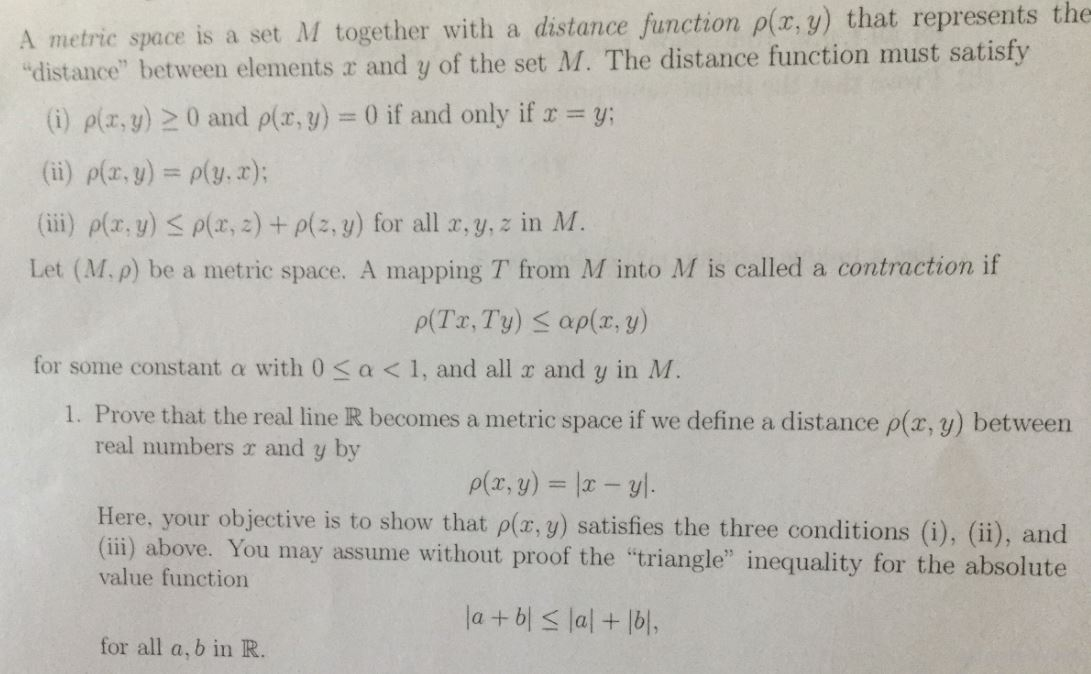# metric space is a set M together with a distance function ρ(x,y) that represents thedistance" between elements a and y of the set M. The distance function must satisfy) plz,y)20 and plr,y) 0 if and only if y:(ii) p(x,y) o(y,z);(iii) p(z, y) a(x, z) +a(z, y) for all x, y, z in M.Let (M.p) be a metric space. A mapping T from M into M is called a contraction iffor some constant a with 0 sa

Question
10 views

pls explain to me step by step. pls dont skip any steps. thankshelp_outlineImage Transcriptionclosemetric space is a set M together with a distance function ρ(x,y) that represents the distance" between elements a and y of the set M. The distance function must satisfy ) plz,y)20 and plr,y) 0 if and only if y: (ii) p(x,y) o(y,z); (iii) p(z, y) a(x, z) +a(z, y) for all x, y, z in M. Let (M.p) be a metric space. A mapping T from M into M is called a contraction if for some constant a with 0 sa<1, and all a and y in M I. Prove that the real line R becomes a metric space if we define a distance ρ(x, y) between real numbers a and y by Here, your objective is to show that p(z. g) satisfies the three conditions (i), (), and (ii) above. You may assume without proof the "triangle" inequality for the absolute value function for all a, b in R fullscreen
check_circle

Step 1

Proof:

The metric defined on R is d (x, y) = |x – y|.

In order to prove the real line is a metric space with given metric, check the three conditions as follows.

To check for the non-negativity of the metric.

Step 2

Check the symmetry property.

Step 3

Check the triangle inequ...

### Want to see the full answer?

See Solution

#### Want to see this answer and more?

Solutions are written by subject experts who are available 24/7. Questions are typically answered within 1 hour.*

See Solution
*Response times may vary by subject and question.
Tagged in

### Math